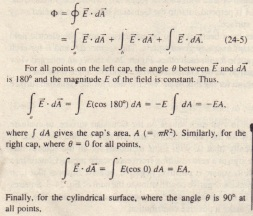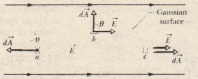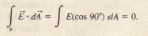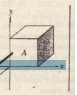# Sample Problem GAUSS LAW

Sample Problem GAUSS LAW

shows a Gaussian surface in the form of a cylinder of  surface radius Rummer ed in a uniform electric field E with the cylinder ax is parallel to the field What is the flux of the electric field through this closed surface  Surface We can do his by writing the flux as the sum of three terms integrals over the left cylinder cap a the cylindrical surface h and the right cap c.A cylindrical Gaussian surface closed by end cap is immersed in a uniform electric fied cylinder axis is parallel to the field direction<I>= -EA + 0 + EA = O.

This result is perhaps not surprising because the field lines that represent the electric field all pass entirely through the Gaussian surface entering through the left end cap leaving through the right end cap and giving a net flux of zero CHECKPOINT  The figure here shows a Gaussian cube of face area A immersed in a uniform electric field E that has the positive direction of the z axis In terms of E and A what is the flux through (a) the front face (which is in they plane rear face(c) E the top face and (d) the whole cube.